# DTFT of a Sampled Sequence

July 24, 2019

Suppose there is a signal x(t) with a Fourier transform of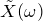. The signal is sampled every 𝜏 seconds, yielding the sequence y(n) = x(n𝜏). From the sampled sequence, a calculation yields the discrete-time Fourier transform (DTFT)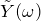of y(n).

Both a Fourier transformand a DTFThave been identified. They come from two different transform definitions but originated from the same signal, x(t).

This prompts the question: doandlook anything alike? The answer is yes; in fact,looks like a scaled and repeated version of. Below, Theorem 1 and Example 1 offer a more precise description as to why this is.

### Theorem 1

Defining the DTFT of a sampled sequence in terms of the Fourier transform of the sampled waveform.

Let 𝗒(𝑛) ≜ 𝗑(𝑛𝜏) be the sample sequence of a waveform 𝗑(𝑡). 𝖥s = 1/𝜏 is the sample rate. Let 𝖸̆(𝜔) be the DTFT of 𝗒(𝑛) and 𝖷̃ (𝜔) be the Fourier transform of 𝗑(𝑡). Then:

(1)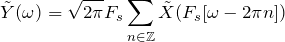#### Proof

This theorem is difficult to prove without resorting to an “engineering hack” methodology such as multiplying the waveform by a train of impulse functions. However, the proof is manageable when we leverage the somewhat obscure yet powerful inverse Poisson summation formula (IPSF).

 (2)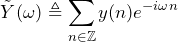by definition of DTFT (3)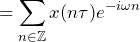by definition of y(n) (4)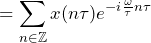because τ/τ = 1 (5)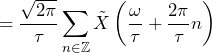by IPSF (6)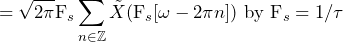and absolute summability

The DTFT of the sample sequence is the Fourier transform of the original waveform. It is:

1. Repeated every 2𝜋 radians (in discrete world frequency, which is every 2 × 𝖥s Hz in real-world frequency)
2. Dilated (made narrower) by a factor of 𝖥s
3. Scaled (made taller) by a factor of √2𝜋𝖥s

Figures 2.1-2.4 illustrate the extraction of the frequency content of a sampled waveform with a sample rate of F= 4 x fh.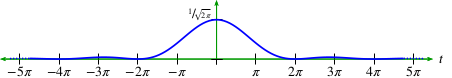Figure 2.1. Time waveform x(t).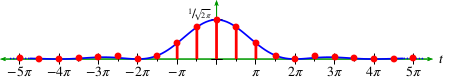Figure 2.2. Sample sequence.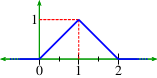Figure 2.3. Fourier transform.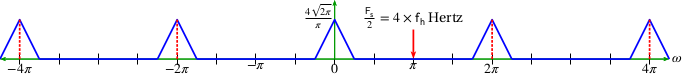Figure 2.4. DTFT of y(n).

Examples 4 through 7 in this lesson and those that follow illustrate the importance of Theorem 1.

### Frequency Content of a Sampled Waveform (Ex. 4)

Fs = 4 x fh

Given the time waveform illustrated in Figure 2.1:

(7)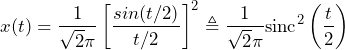The Fourier transform 𝖷̃ (𝜔) is the triangle function illustrated in Figure 2.3. The highest frequency component is 𝜔h = 1 radian/second or 𝖿h = 1/2𝜋 Hz. Therefore, the minimum sample rate is 𝖥s ≥ 2 × 𝖿h = 1/𝜋 and the maximum sample period is 𝜏 ≤ 𝖥s = 𝜋.

The waveform 𝗑(𝑡) sampled at 𝖥s ≜ 4 × 𝖿h = 4 × 1/2𝜋 = 2/𝜋 (giving a sample period of 𝜏 = 1/𝖥s = 𝜋/2) is illustrated in Figure 2.2.

With this sample rate and Theorem 1, the DTFT 𝖸̃(𝜔) of 𝗒(𝑛) is a repeated and scaled version of 𝖷̃ (𝜔). It is expressed as:

(8)(9)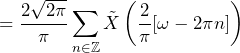### Conclusion

• 𝖷̃ (𝜔) repeats every 2𝜋 (this is true of all DTFTs by definition)
• 𝖷̃ (𝜔) reaches zero when (2/𝜋) 𝜔 = 1 or 𝜔 = 𝜋/2; the higher the sample rate 𝖥s, the narrower the triangle illustrated in Figure 2.3
• The height of each triangle is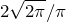or about 1.596. The higher the sample rate 𝖥s, the higher the triangle illustrated in Figure 2.3